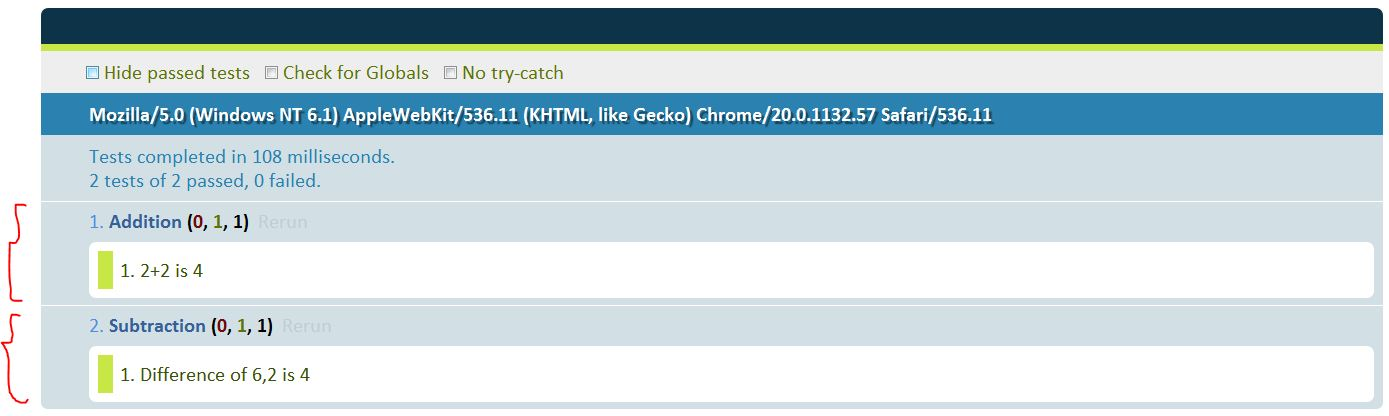# Unit testing JavaScript – II

In the earlier post, we discussed the need for unit testing JavaScript code. Continuing further, let’s write a simple piece of JavaScript code and test it using QUnit library.

To begin with, let’s create a file calculator.js and have two functions add and subtract as shown below. You can ignore the UI for the time being.

```function add(a,b){
return a+b;
}
function subtract(a,b){
return a-b;
}
```

Let’s create a calculatortests.js file where we’ll use QUnit to test the add and subtract functions. QUnit provides simple syntax to test the functions. We’ll use the simple function “equal” for asserting the results of add and subtract functions. The code to test the add and subtract functions are shown below.

```test("Addition",function(){
});
test("Subtraction",function(){
equal(4,subtract(6,2),"Difference of 6,2 is 4");
});
```

Every test runs independently similar to the unit testing nomenclature. The test function accepts the name of the test and a function that is executed as the test. The equal function is similar to the assertXXX methods in JUnit. That’s it!!! Let’s set up the whole stuff and execute the tests.

Let’s create a calculatortests.html with the the following files included, qunit.css, qunit.js, calculator.js, and calculatortests.js. For QUnit to publish the results in the page we need the following div element in the html file.

```<div id="qunit"></div>
```

The calculatortests.html file is shown below.

```<!DOCTYPE html>
<html>
<script src="qunit-1.9.0.js"></script>
<script src="calculator.js"></script>
<script src="calculatortests.js"></script>